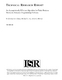## An Asymptotically Efficient Algorithm for Finite Horizon Stochastic Dynamic Programming Problems##### Files
TR_2003-26.pdf(252.94 KB)
We present a novel algorithm, called Simulated Annealing Multiplicative Weights", for approximately solving large finite-horizon stochastic dynamic programming problems. The algorithm is asymptotically efficient" in the sense that a finite-time bound for the sample mean of the optimal value function over a given finite policy space can be obtained, and the bound approaches the optimal value as the number of iterations increases.The algorithm updates a probability distribution over the given policy space with a very simple rule, and the sequence of distributions generated by the algorithm converges to a distribution concentrated only on the optimal policies for the given policy space. We also discuss how to reduce the computational cost of the algorithm to apply it in practice.